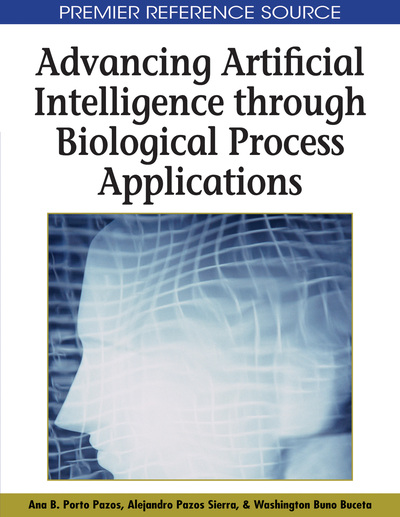# A Genetic Algorithm-Artificial Neural Network Method for the Prediction of Longitudinal Dispersion Coefficient in Rivers

Jianhua Yang (University of Warwick, UK), Evor L. Hines (University of Warwick, UK), Ian Guymer (University of Warwick, UK), Daciana D. Iliescu (University of Warwick, UK), Mark S. Leeson (University of Warwick, UK), Gregory P. King (University of Warwick, UK) and XuQuin Li (University of Warwick, UK)
DOI: 10.4018/978-1-59904-996-0.ch019
Available
\$37.50
No Current Special Offers

## Abstract

In this chapter a novel method, the Genetic Neural Mathematical Method (GNMM), for the prediction of longitudinal dispersion coefficient is presented. This hybrid method utilizes Genetic Algorithms (GAs) to identify variables that are being input into a Multi-Layer Perceptron (MLP) Artificial Neural Network (ANN), which simplifies the neural network structure and makes the training process more efficient. Once input variables are determined, GNMM processes the data using an MLP with the back-propagation algorithm. The MLP is presented with a series of training examples and the internal weights are adjusted in an attempt to model the input/output relationship. GNMM is able to extract regression rules from the trained neural network. The effectiveness of GNMM is demonstrated by means of case study data, which has previously been explored by other authors using various methods. By comparing the results generated by GNMM to those presented in the literature, the effectiveness of this methodology is demonstrated.
Chapter Preview
Top

## Introduction

An important application of environmental hydraulics is the prediction of the fate and transport of pollutants that are released into watercourses, either as a result of accidents or as regulated discharges. Such predictions are primarily dependent on the water velocity, longitudinal mixing, and chemical/physical reactions etc, of which longitudinal dispersion coefficient is a key variable for the description of the longitudinal spreading in a river.

The concept of longitudinal dispersion coefficient was first introduced in Taylor (1954). Based on this work, the following integral expression was developed (Fischer, List, Koh, Imberger, & Brooks, 1979; Seo & Cheong, 1998) and generally accepted:

(1) where K = longitudinal dispersion coefficient; A = cross-sectional area; B = channel width; h = local flow depth; u’ = deviation of local depth mean flow velocity from cross-sectional mean; y = coordinate in the lateral direction; and εt = local (depth averaged) transverse mixing coefficient. An alternative approach utilises field tracer measurements and applies the method of moments. It is also well documented in the literature (Guymer, 1999; Rowinski, Piotrowski, & Napiorkowski, 2005; Rutherford, 1994) and defines K as (2) where Uc = mean velocity, x1 and x2 denotes upstream and downstream measurement sites,= centroid travel time, σt2(x) = temporal variance,

However, owing to the requirement for detailed transverse profiles of both velocity and cross-sectional geometry, equation (1) is rather difficult to use. Furthermore, equation (2), called the method of moments (Wallis & Manson, 2004), requires measurements of concentration distributions and can be subject to serious errors due to the difficulty of evaluating the variances of the distributions caused by elongated and/or poorly defined tails. As a result, extensive studies have been made based on experimental and field data for predicting the dispersion coefficient (Deng, Singh, & Bengtsson, 2001; Jobson, 1997; Seo & Cheong, 1998; Wallis & Manson, 2004).

For example, employing 59 hydraulic and geometric data sets measured in 26 rivers in the United States, Seo and Cheong (1998) used dimensional analysis and applied the one-step Huber method, a nonlinear multi-regression method, to derive the following equation:

(3) in which u* = shear velocity. This technique uses the easily measureable hydraulic variables of B, H and U, together with a frequently used parameter, extremely difficult to accurately quantify in field applications, u*, to estimate the dimensionless dispersion coefficient K from equation (3). Another empirical equation developed by Deng et al. (2001) is a more theoretically based approximation of equation (1), which not only includes the conventional parameters of (B/H) and (U/u*) but also the effects of the transverse mixing εt0, as follows: (4) where

(5)

## Complete Chapter List

Search this Book:
Reset